# Discussing the Importance of Stationary Residuals in Time Series

A traditional approach of analyzing the residuals in regression models can be identified over the Classical Assumptions in Linear Models (Rodríguez Revilla, 2014), which primarily involves the residuals in aspects as homoscedasticity, no serial correlation (or auto-correlation), no endogeneity, correct specification (this one includes no omitted variables, no redundant variables, and correct functional form) and finally, normal distribution among the estimated residuals of the model with expected zero mean.

In time series context, residuals must be stationary in order to avoid spurious regressions (Woolridge, 2012), if there are no properties of
stationarity among the residuals, then basically our results tend to produce
fake relationships in our model. At this point, it is convenient to say:

“A stationary time series
process is one whose probability distributions are stable over time in the
following sense: if we take any collection of random variables in the sequence
and then shift that sequence ahead h times periods, the joint probability
distribution must remain unchanged”
(Woolridge, 2012, pág. 381)

Another definition according to Lutkepohl & Kratzig (2004) says that stationarity has time-invariant first and second moments over a single variable, mathematically: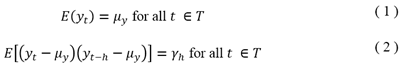Equation (1) simply implies that the expected values of the y process must have a constant mean, so the stationary process must fluctuate around a constant mean defined in µ, no trends are available in the process. Equation
(2) is telling us that variances are time-invariant, so the term γ, doesn’t depend on t but just on the distance h.

In order to get a better notion of stationarity, we define that a stationary process follows the pattern in the next graph. Which was generated using random values over a constant mean of 0, and with a normal probability distribution. The time period sample was n=500 observations.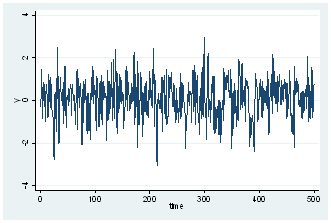The generated process fluctuates around a constant mean, and no tendency is present. How do we confirm if the series is normally distributed? Well, we can perform a histogram over the series. In Stata, the command is histogram y, norm where y is our variable.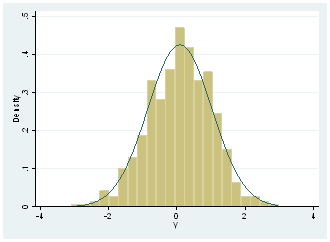The option of ,norm is given in Stata in order to present the actual normal distribution, so we can see that real distribution it’s not far from it. We can graphically affirm that series might present a normal distribution, but in order to confirm it, we need to do a formal test, so we perform Jarque-Bera test with the command sktest y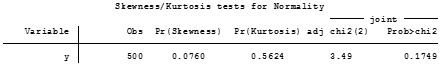The null hypothesis of the test is that normal distribution exists among the y variable And since p-value is bigger than a 5%significance level, we fail to reject null hypothesis and we can say that y variable is normally distributed.

Checking for unit roots also is useful when we’re trying to discover stationarity over a variable, so we perform first, the estimated ideal lag for the test, with varsoc y which will tell us what appropriated lag-length should be used in the ADF test.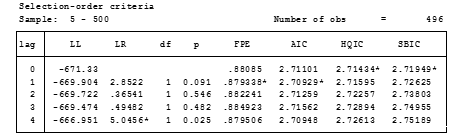Such results, indicate that ADF test over y variable must be done with one lag according to FPE, AIC, while HQIC and SBIC indicate 0 lags. It is the decision of the investigator to select the right information criteria (mostly it is selected when all error criteria are in a specific lag). However, we have a draw of FPE and AIC vs HQIC and SBIC. We will discard FPE since according to Liew (2004) this one is more suitable for samples lower than 120 observations, and thus we will select 0 lag for the test considering our sample size of 500 observations.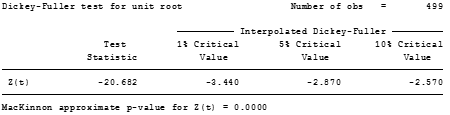Null hypothesis is the existence of unit roots in the variable, so we can strongly reject this and accept that no-unit roots are present. Sometimes this test is used to define stationarity of a respective process, but we need to take in consideration that stationarity involves constant means and normal distributions. We can say for now, that y variable is stationary.

At this point, one could argue Why we need the notion of stationarity over the residuals? This is because stationarity ensures that no spurious regressions are estimated. Now let’s assume we have a model which
follows an I (0) stationary model.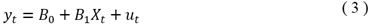And that I (0) variables are y and x, common intuition will tell us that u will be also stationary, but we need to ensure this. Proceeding with our Monte Carlo approaches, we generated the x series with a constant mean which has a normal distribution and that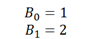with u ~ (0,1) as the Data Generating Process of y expressed in equation (3). Basically u has a mean of 0, and variance of 1. Regressing y on x we got the next result.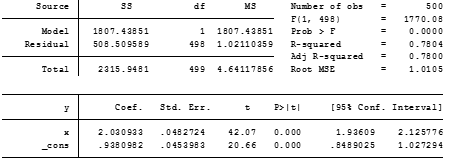We can see that coefficients B_0 and B_1 are approximated 1 and 2 respectively, so it’s almost close to the data generating process and both estimators are statistically significant at 1%. Let’s look at the residuals of the estimated model a little bit closer, we start by predicting the residuals using the command predict u, residuals in order to get the predicted values. Then we perform some of the tests we did before.

Graphic of the residuals with tsline u presentsthe next result, which looks like a stationary process.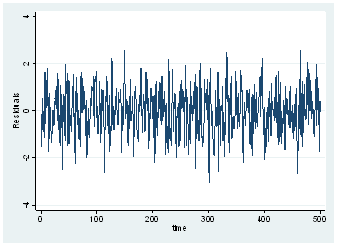A histogram over the residuals, will show the pattern
of normal distribution.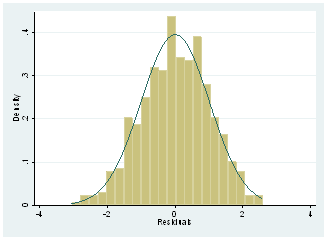And as well, the normality test will confirm this result.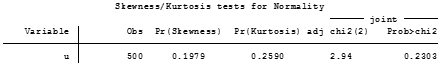Now we need to test that the residuals don’t follow a unit root pattern, a consideration here must be done first before we use ADF test, and is that critical values of the test are not applicable to the residuals. Thus, we cannot fully rely on this test.

In Stata we can recur to the Engle-Granger distribution test of the residuals, to whether accept or reject the idea that residuals are stationary. So, we type egranger y x which provides an accurate estimate of the critical values to evaluate the residuals.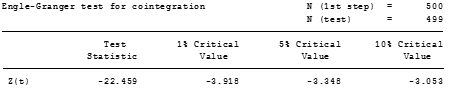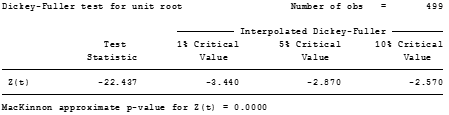As tests evidence, Test statistic is pretty close between ADF test and Engle & Granger test but the critical values are way different. Furthermore, we should rely on the results of the Engle & Granger test. Since Test statistic is bigger than 5% critical value, we can reject the null hypothesis that x and y are not cointegrated, and we can affirm that both variables present over this estimation a long run path of equilibrium. From another view, implies that the residuals are stationary and our regression is not spurious.

This basic idea can be extended with I (1) variables, in order to test whether it exists a long run path and if the regression model in (3) turns to be super consistent. Then long-run approximations with error correction forms can be done for this model where all variables are I (1).

This idea of testing residuals in stationary models is not a formal test used in the literature, however, it can reconfirm that with I (0) models that the regression will not be spurious. And it can also help to contrast long-run relationships.

Note: The package egranger must be installed first ssc install egranger, replace should do the trick. This package parts from the regression model to be estimated, however, it has the failure it cannot be computed with time operators. So, generating first differences or lagged values must be done in separate variables.

Bibliography

Liew, V. (2004). “Which Lag Length Selection Criteria Should We Employ?”. Journal of Economics Bulletin, 1-9. Recuperated from:

Lutkepohl, H., & Kratzig, M. (2004). Applied Time Series Econometrics. Cambridge: Cambridge university press.

Rodríguez Revilla, R. (2014). Econometria I y II. Bogotá. : Universidad Los

Woolridge, J. (2012). Introductory Econometrics. A Modern Approach 5th edition. United States: South Western Cengage Learning.

### 7 thoughts on “Discussing the Importance of Stationary Residuals in Time Series”

1.It is amazing and wonderful to visit your site. Thanks for sharing information; this is useful to us….

2.Pretty! This was a really wonderful article. Thank you for supplying this info.

3.I like the valuable information you supply for your articles.
I will bookmark your blog and test once more right here frequently.
I’m somewhat certain I will be informed many new stuff right right
here! Best of luck for the following!

4.Some truly nice stuff on this internet site , I like it.

5.360DigiTMG_vizag

Really nice and interesting post. Keep posting. Thanks for sharing.

360DigiTMG

6.This post really too helpful for me to do blog commenting. Thank you for sharing